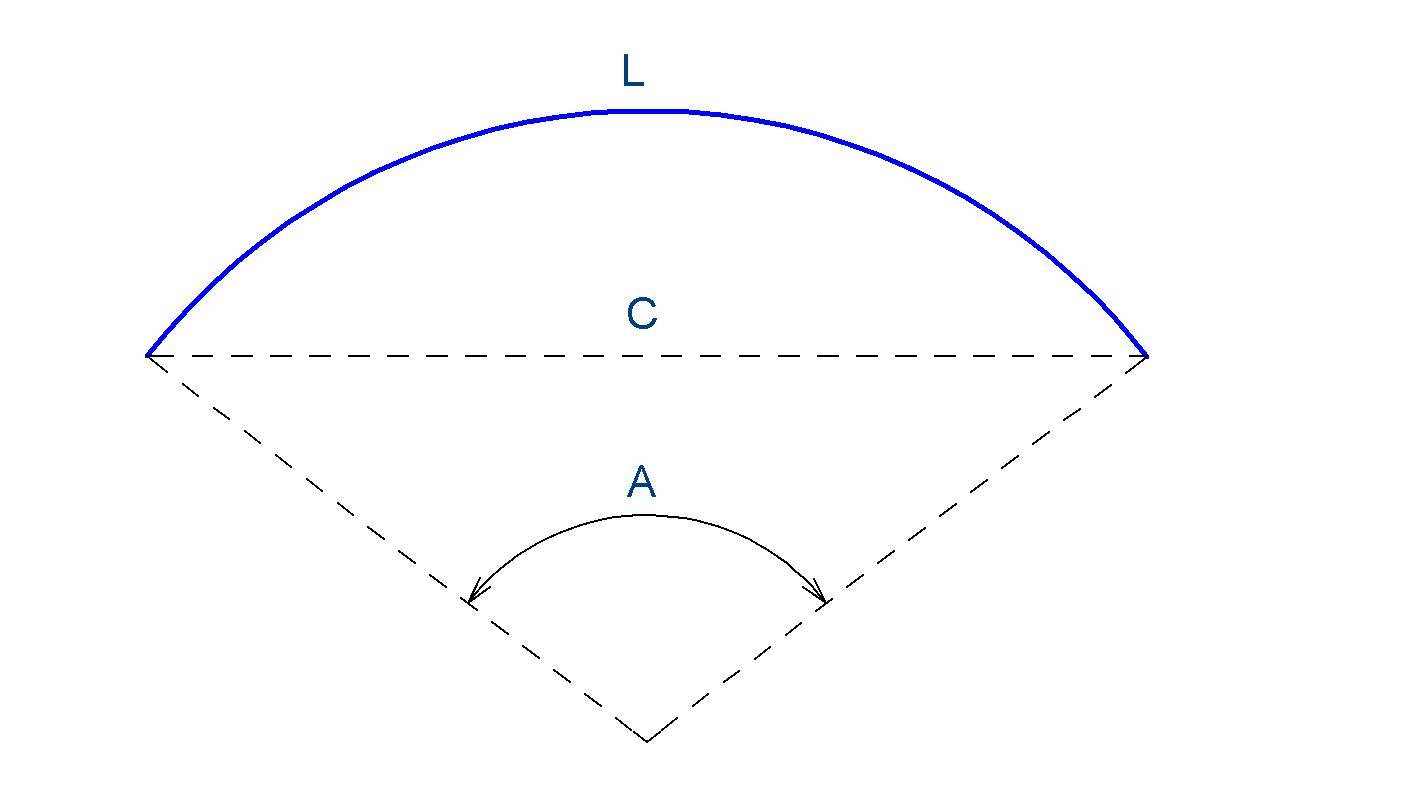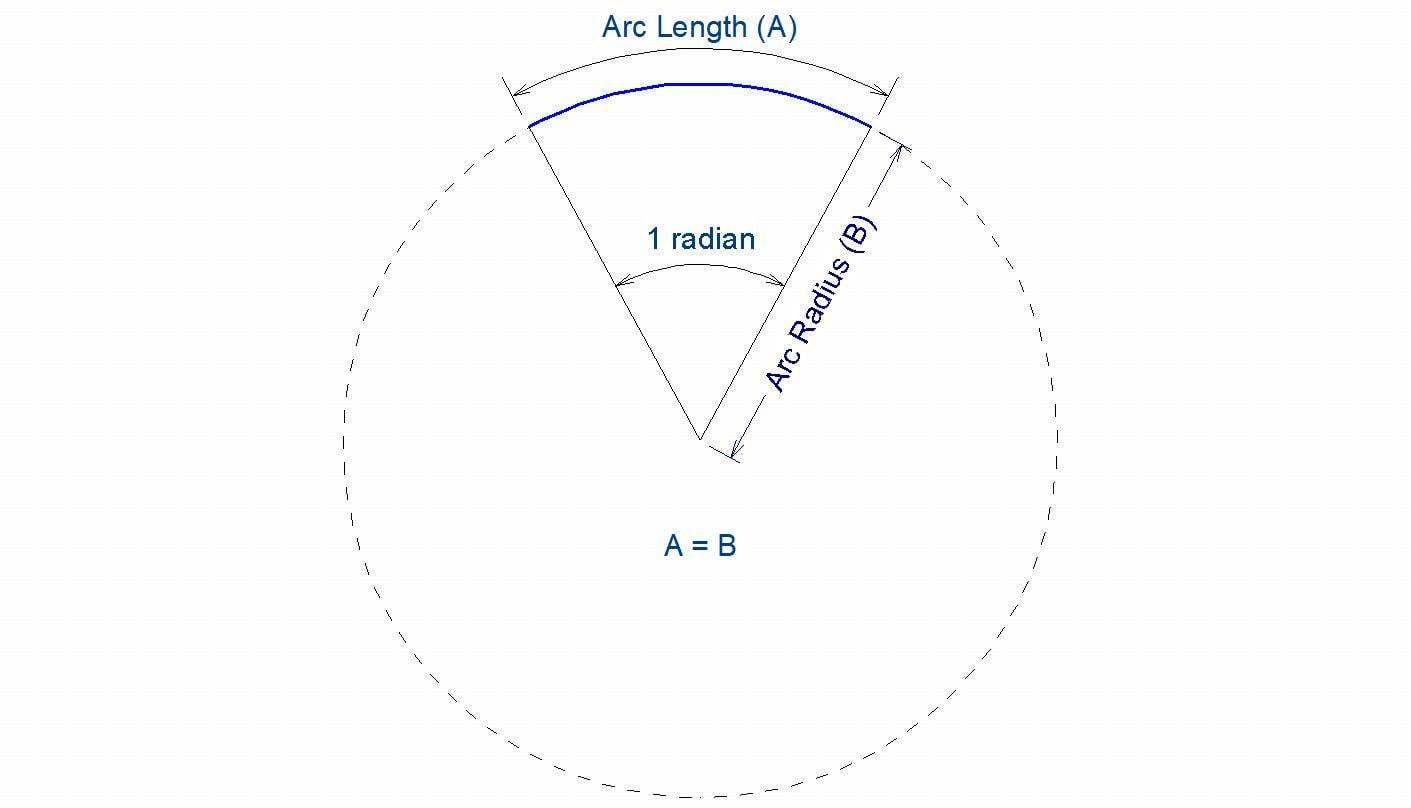# Formula to find arc radius using arc length, chord length, and/or segment angle

• MHB
In summary, @Klaas van Aarsen explained that the angle A in radians is the arc length L divided by the radius R.f

I'm attempting to write some code using the Ruby programming language that will give me the radius of an arc but the only pieces of information I have to work with are the arc length (L), the chord length (C), and the circular segment angle in degrees (A):I'm hoping someone can show me how to insert L, C, and A into a formula that I can use in my code. I'm working with a CAD program that opens up access to certain pieces of information about each arc, but unfortunately radius isn't one of them, so I need to use the information I do have to find the radius.

Thanks so much for your time.

Last edited:
Let R be the radius of the arc.
Then the angle A in radians is defined as the arc length L divided by the radius R.
In other words:
$$A = \frac L R\implies R=\frac{L}{A}$$

Let R be the radius of the arc.
Then the angle A in radians is defined as the arc length L divided by the radius R.
In other words:
$$A = \frac L R\implies R=\frac{L}{A}$$

@Klaas van Aarsen Thank you! I was able to use your answer to write my desired code section. I just needed to add some language to convert degrees to radians (degrees*pi/180). My finished section of Ruby code looks like this:
Ruby:
arc_length/(arc_angle * Math::PI / 180)

Thanks so much for the help : )

Pretty elementary I know, but just in case this helps anyone else:
I feel a little silly in retrospect. I searched all over the place online before posting here. My problem was that I didn't until today understand what a radian was. A radian is essentially defined as the circular segment angle at which the arc length is equal to the radius. I've attempted to illustrate this in the image below:For me personally, I can then pretty easily go through all the logic like so:
• As most people know, a circle's circumference can be measured by its radius*2*pi, therefore...
• A full circle contains a quantity of radians that can be represented as 2*pi, therefore...
• 360 degrees/(2*pi) or 180/pi gives me the number of degrees in a radian, therefore...
• The inverse of 180/pi or pi/180 will give me the number of radians in a degree
• Knowing my degrees, I therefore know exactly how many radians I'm working with...
• Knowing my arc length, I can then simply divide that by my number of radians to get my radius
Its all quite easily understandable using things I already knew as soon as I understood what a radian was. Thanks again @Klaas van Aarsen for giving me an answer that got me looking in the right place.

Last edited:
Its funny, it just occurred to me that it was right there in front of me all along. I didn't even need to know what a radian was at all. Its really more of a concept than it is a necessary number. In and of itself its pretty meaningless. It all just came back to what I learned way back in grade school:
diameter*pi = circumference. I could have easily extrapolated everything I needed from that had I thought about it a little harder.